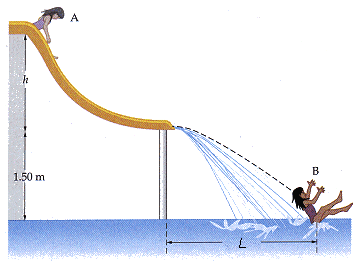# Projectiles and conservation of energy (water slide problem)

#### wmmk

1. The problem statement, all variables and given/known data
The water slide shown in the figure ends at a height of 1.50 m above the pool. If the person starts from rest at point A and lands in the water at point B, which has a horizontal distance L = 2.58 m from the base of the slide, what is the height h of the water slide? (Assume the water slide is frictionless.)2. Relevant equations
PEi+KEi+W=PEf+KEf
PE=mgh
KE=(1/2)mv2
Vertical displacement after leaving slide: Dy=vot+(1/2)at2
Horizontal displacement after leaving slide Dx=vft

3. The attempt at a solution
PEi+KEi+W=PEf+KEf
PEi=KEf
mghi=(.5)mvf2
(9.81)h=(.5)vf2

I'm not really sure where to go from here. Any help would be greatly appreciated.
Thanks,
Will

#### rock.freak667

Homework Helper
well v would be v=√(2gh).

It looks like the person leaves the slide horizontally. Use the kinematic equations, you are given the range L.

#### kjohnson

First lets consider the energy part of the problem by setting up an coordinate frame such that y=0 at the bottom of the slide. From this you can apply the conservation of mechanical energry theorm PE1+KE1=PE2+KE2 which will result in PE1=KE2. This is because we defined y=0 at the base of the slide and thus PE2=0 with respect to that frame. Now lets look at the projectile part of the problem. We know how far the person traveled in the 1.5m from the ground to the base of the slide, and we can calcualte the time it takes an object to fall 1.5 under the influence of gravity. So we know distance in the x direction and the time it took to travel that distance, thus we know our x velocity Vx. Last we link the energy and projectile portions of the problem to obtain a solution. We can now do this because we know KE2 since we calculated that velocity Vx. If we know KE2 we know the potential energy PE1 at the top of the slide and thus the height.

#### kjohnson

Also note that work W should not appear in the conservation of mechanical energy equation. If you were refering to Wg the work due to gravity you doubled down on that term since Wg is already accounted for in terms of potential energy in the conservation of mechanical energy equation.

### The Physics Forums Way

We Value Quality
• Topics based on mainstream science
• Proper English grammar and spelling
We Value Civility
• Positive and compassionate attitudes
• Patience while debating
We Value Productivity
• Disciplined to remain on-topic
• Recognition of own weaknesses
• Solo and co-op problem solving